Varsity Tutors always has a different Calculus 1 Question of the Day ready at your disposal! If you’re just looking to get a quick review into your busy day, our Calculus 1 Question of the Day is the perfect option. Answer enough of our Calculus 1 Question of the Day problems and you’ll be ready to ace the next test. Check out what today’s Calculus 1 Question of the Day is below.

### Question of the Day: Calculus 1

If the acceleration of an object is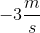, and its acceleration function is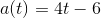, what is the velocity of the object?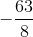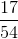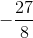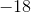Calculus 1 is one of the more advanced subjects of math, prior to Calculus 2 and 3. If you need some help in getting the concepts down, you may benefit from using Varsity Tutors’ Learning Tools. You can easily access daily Calculus 1 practice, like the Question of the Day. With this tool, you are given random questions inspired by the questions on major Calculus 1 exams. You can work closely with important concepts, and receive regular, free practice. Calculus 1 is an important precursor as you get into more advanced mathematical concepts. By 11th or 12th grade, you may be taking Calculus 1, particularly if you are planning to go into physics, or other careers that require high-level math. Whether you need Calculus tutoring in Atlanta, Calculus tutoring in Houston, or Calculus tutoring in San Francisco, working one-on-one with an expert may be just the boost your studies need.

Once a day, the Question of the Day is chosen randomly from the vast amount of Calculus 1 material included in the Learning Tools. Through daily practice, the Question of the Day can keep information fresh in your mind. You are able to get daily test practice without dealing with the usual stressors that come along with a real test. The questions offer detailed analysis on your current knowledge through the results, helping you determine where to focus your studies and create the study plan that works best for you. Varsity Tutors also offers resources like free Calculus 1 Practice Tests to help with your self-paced study, or you may want to consider an Calculus 1 tutor.

With the Calculus 1 Question of the Day, you are quizzed on the different concepts behind the subject. You may see questions on finding the midpoint Riemann sums, differential functions, local maximums, integrals, graphing areas and curves, intervals, function meanings, rate approximation, regional math, and spatial calculus, such as velocity, distance, and acceleration. You will be tested on how you find differentiable rates, making prediction models, and identifying the rate of change. Each of these concepts is later used in Calculus 2 and 3, just as your basic math concepts tie into Calculus 1.

When you answer the daily question, the Learning Tools offer you a detailed analysis of the answer. You are given a percentile, which is based on the number of people that answered the question that day. In addition, the analysis lists the concept title, and an explanation behind the reason for the answer. You can not only see where you make mistakes, but also the reason behind the correct answer, which is often what you need in order to improve your skills. In addition to the Calculus 1 Question of the Day and Calculus tutoring, you may also want to consider taking some of our Calculus 1 Flashcards.

The various Learning Tools can be used individually or together. When you create your Calculus 1 study plan, you can incorporate several of the Learning Tools to focus on different aspects of the subject, such as flashcards to help you memorize information, or the Learn by Concept interactive syllabus to learn more about specific concepts. If you need extra Calculus 1 help, you can help yourself with Varsity Tutors’ Learning Tools. You can use them alongside your coursework, strengthening the information you are taught on a daily basis, or you can use them for individual study periods. When you have the right tools, you can prepare for the challenges of Calculus 1 tests with ease.

### All Calculus 1 Resources